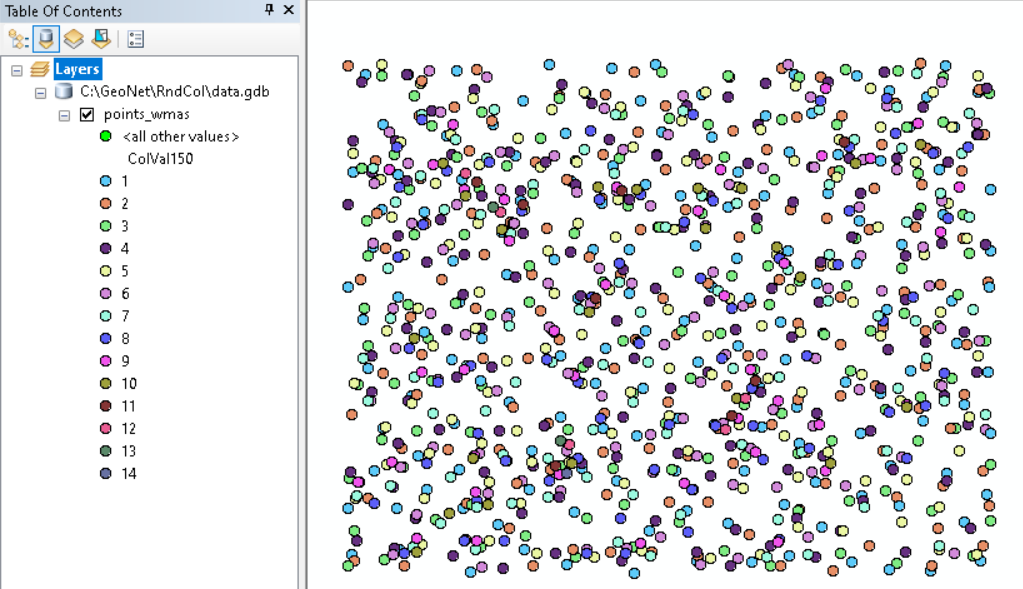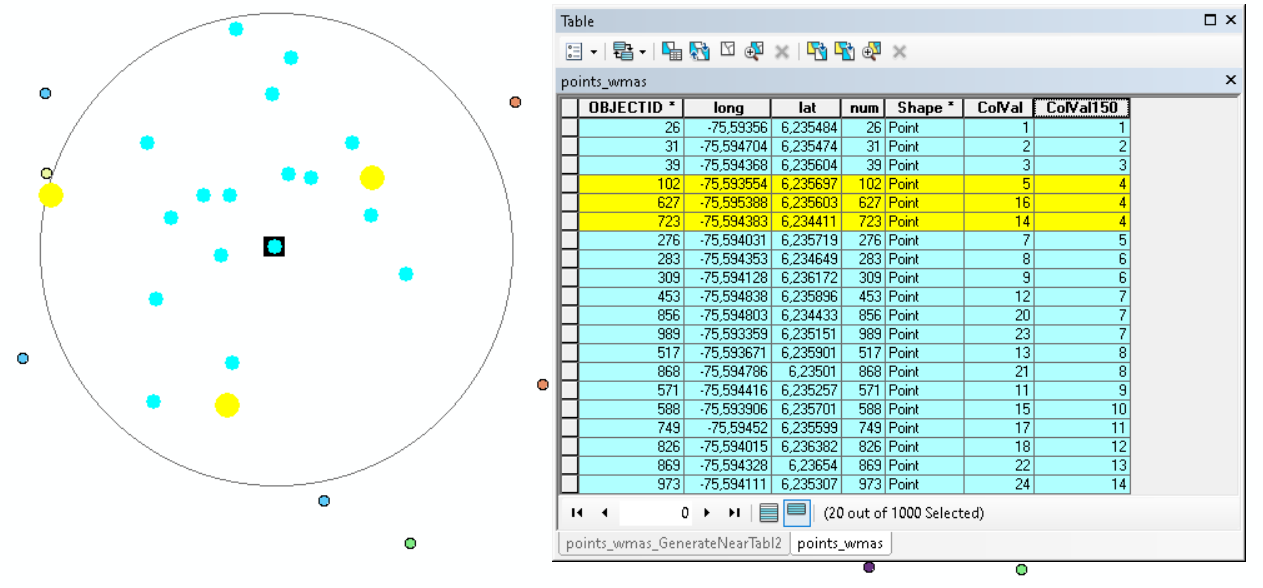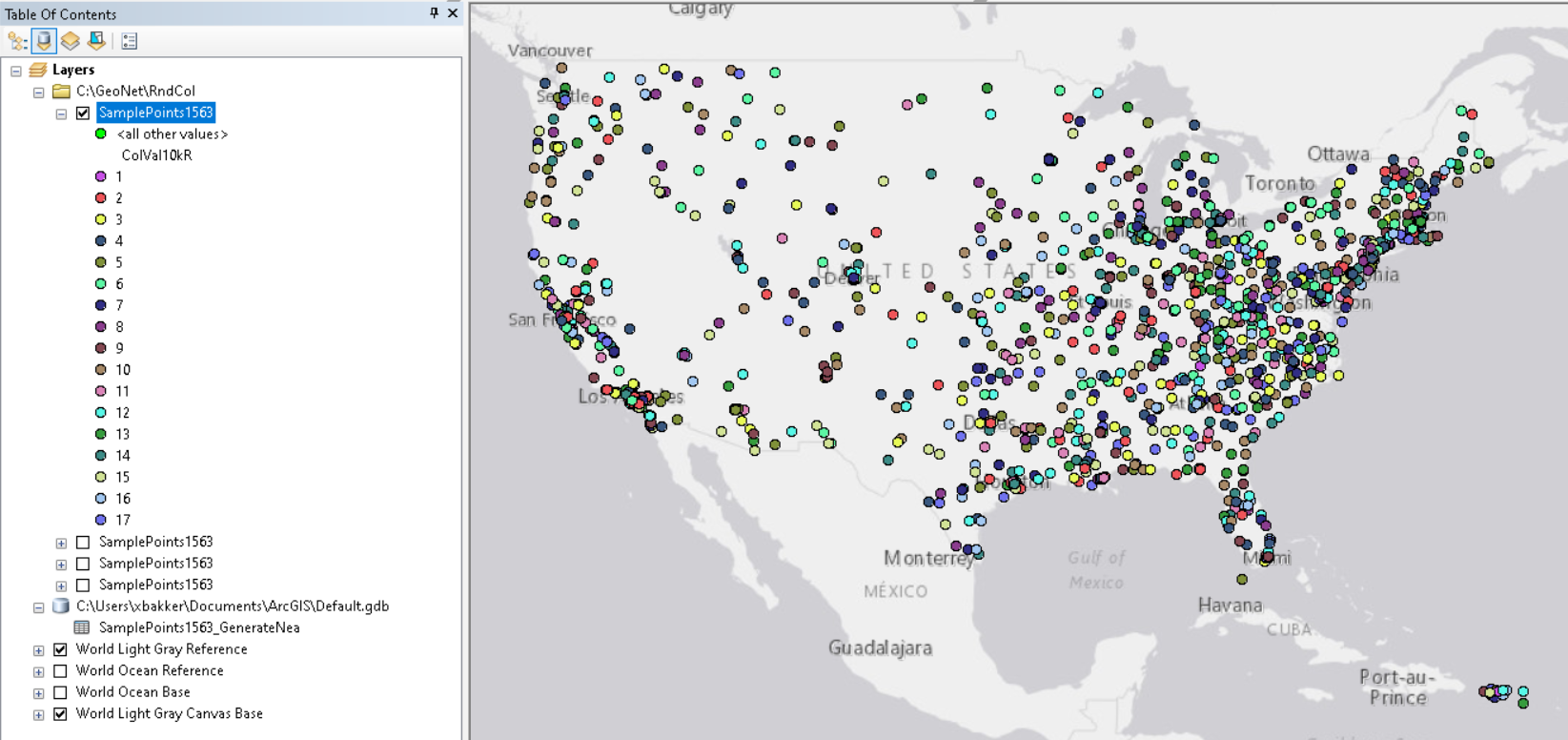# Apply symbology based on an algorithm

928
10
08-28-2019 01:08 PMNew Contributor III

Been battling this problem for a long time now, so hoping someone here can shed light on a possible solution. The problem is that I have 100 points and I want to apply a color palette to the points so that no two points within 25 miles have the same color. The issue I'm trying to prevent is having points close to each other being mistaken for similarity.

I think I'm getting somewhere with running the Generate Near Table in ArcGIS Pro 2.3 which will find all near points within 25 miles. Conceptually, I'm thinking this could turn into a Python script or ModelBuilder model where a first point is selected, Color field is given a value, then it moves on to the next record, but it can't be from a list of OIDs found in the Near Table result for that next record.

To recap, I'm trying to apply, for example, 10 colors to 100 points, where no points within 25 miles of each other gets the same color applied. Some code that I've been trying to play around with:

``````import arcpy

# Process: Select Layer By Attribute
arcpy.SelectLayerByAttribute_management('InputPoints100', 'NEW_SELECTION',"OBJECTID = 1")

# Process: Calculate Field

arcpy.Append_management('InputPoints100', 'InputPoints_LIST', 'TEST')

################################################################# ONE ######################################################################
arcpy.SelectLayerByLocation_management('InputPoints100', 'WITHIN_A_DISTANCE', 'InputPoints100',"25 Miles", 'NEW_SELECTION', 'INVERT')

layer = "InputPoints100"
count = 1

def randomColor():

def SelectRandomByCount (layer, count):
import random
layerCount = int (arcpy.GetCount_management (layer).getOutput (0))
if layerCount < count:
print("input count is greater than layer count")
return
oids = [oid for oid, in arcpy.da.SearchCursor (layer, "OID@")]
oidFldName = arcpy.Describe (layer).OIDFieldName
randOids = random.sample (oids, count)
oidsStr = ", ".join (map (str, randOids))
sql = "{0} IN ({1})".format (delimOidFld, oidsStr)
arcpy.SelectLayerByAttribute_management (layer, "", sql)

SelectRandomByCount(layer, count)

arcpy.Append_management('InputPoints100', 'InputPoints_LIST', 'TEST')

with arcpy.da.SearchCursor(layer,field_names = "ObjectID") as cursor:
for row in cursor:
randomColor()

print("Done!")‍‍‍‍‍‍‍‍‍‍‍‍‍‍‍‍‍‍‍‍‍‍‍‍‍‍‍‍‍‍‍‍‍‍‍‍‍‍‍‍‍‍‍‍‍‍‍‍‍‍‍‍‍‍‍‍‍‍‍‍‍‍‍‍‍‍‍‍‍‍‍‍‍‍‍‍‍‍‍‍‍‍‍‍‍‍``````
Tags (3)
10 RepliesbyEsri Esteemed Contributor

This seems to work:

``````def main():
import arcpy

fc = r'C:\GeoNet\RndCol\data.gdb\points_wmas'
fld = 'ColVal150'
search_dist = 150.0

# create dictionaries
print("create dictionaries...")
flds = ("OID@", "SHAPE@")
dct_shp = {r: r for r in arcpy.da.SearchCursor(fc, flds)}
flds = ("OID@", fld)
dct_col = {r: r for r in arcpy.da.SearchCursor(fc, flds)}

# loop through features
print("loop through features...")
oids = sorted(dct_col.keys())
for oid in oids:
if oid % 100 == 0:
print(" - {}".format(oid))
pntg = dct_shp[oid]
oids_near = GetNearPointsOIDs(dct_shp, pntg, search_dist)
vals_in_use = GetValuesInUse(oids_near, dct_col)
first_val = GetFirstValue(vals_in_use)
dct_col[oid] = first_val

# write results
print("write results...")
flds = ("OID@", fld)
with arcpy.da.UpdateCursor(fc, flds) as curs:
for row in curs:
oid = row
row = dct_col[oid]
curs.updateRow(row)

def GetNearPointsOIDs(dct_shp, pntg, dist):
oids = []
for oid, pntg_i in dct_shp.items():
if pntg.distanceTo(pntg_i) <= dist:
oids.append(oid)
return oids

def GetValuesInUse(oids, dct_col):
values = []
for oid in oids:
val = dct_col[oid]
if not val is None:
values.append(val)
return values

def GetFirstValue(vals_in_use):
val = None
if len(vals_in_use) == 0:
val = 1
else:
i = 0
while val is None:
i += 1
if not i in vals_in_use:
val = i
return val

if __name__ == '__main__':
main()‍‍‍‍‍‍‍‍‍‍‍‍‍‍‍‍‍‍‍‍‍‍‍‍‍‍‍‍‍‍‍‍‍‍‍‍‍‍‍‍‍‍‍‍‍‍‍‍‍‍‍‍‍‍‍‍‍‍‍‍‍‍‍‍‍‍‍``````

Basically what it does is for every feature search the near features within a defined search distance and check which values are already in use and assign the lowest not used value to this feature.New Contributor III

I think I understand what your code is doing, but when I use it on my data, I get most of the points with a value of '1', some with '2' and then one or two records each of 3, 4, 5, 6, 7, 8, 9. The result I see doesn't show that it's accounting for distance either. In your code, you set a distance of '150.0', but what unit are you using?

The code runs without error on a sample set of 100 points and also on a sample set of 1400 points, but the result is the same. Is there anything other than 'fc' and 'fld' that I would have to change in order for this to run like your screenshot?byEsri Esteemed Contributor

You will need to change the search_dist, whiich in my case is set to 150 map units (meters for me).  The larger the search distance, the higher the assigned values will be. You will have to set your distance to 25 miles and express it in the map units you are using. If you are using a geographic coordinate system, you will have to project your data or change the distanceTo method in the GetNearPointsOIDs function in order to calculate the distance correctly.

The fld variable points to an existing field in your featureclass where the resulting values will be stored.

If you can share your data, I will be able to see what might be going wrong in your case.New Contributor III

Got it, I increased to ~40,000 meters which is around my target of 25 miles, but it's grouping the points into 51 different classes. Is there a way to restrict this to something smaller like 10-15? My ultimate goal is to apply a color palette where users won't be confused by similar shades of the same color, so the way to account for this is to reduce the number of 'ColVal150' classes.byEsri Esteemed Contributor

Hi dtrolling ,

Have a look at the location where the class value 51 was assigned and draw a circle of 25 miles around it to see how many points you have there. It will be more than 51 since values can repeat within that circle as long as they are more than 25 miles apart. The only way to reduce the number of classes is to reduce the search distance.byEsri Esteemed Contributor

Have a look at this example;Within the circle all values 1- 14 are in use, however a couple of them are used multiple times like 4 an 7 (both 3 times) but those are only assigned to points located at larger distances than the search distance.

I just noticed you attached the data. I will have a look at it in a moment.byEsri Esteemed Contributor

Looking at your data is see a lot of points near NYC and there are sparse data in large part of the USA. As you mentioned, most points in those sparse areas will have the value 1 or 2 which makes it very homogeneous and not what you want as I deduce from your comments. To reduce the number of values (colors) you will have to reduce the search distance. I used 10km and that resulted in 17 different values. I also applied a randomizer for those sparse areas to have different colors:The code used for this is listed below:

``````def main():
import arcpy

fc = r'C:\GeoNet\RndCol\data.gdb\points_wmas'
fc = r'C:\GeoNet\RndCol\SamplePoints1563.shp'
fld = 'ColVal10kR' # 'ColVal150'
search_dist = 10000.0 # 150.0

# create dictionaries
print("create dictionaries...")
flds = ("OID@", "SHAPE@")
dct_shp = {r: r for r in arcpy.da.SearchCursor(fc, flds)}
flds = ("OID@", fld)
dct_col = {r: r for r in arcpy.da.SearchCursor(fc, flds)}

# loop through features
print("loop through features...")
oids = sorted(dct_col.keys())
for oid in oids:
if oid % 100 == 0:
print(" - {}".format(oid))
pntg = dct_shp[oid]
oids_near = GetNearPointsOIDs(dct_shp, pntg, search_dist)
vals_in_use = GetValuesInUse(oids_near, dct_col)
first_val = GetFirstValue(vals_in_use)
dct_col[oid] = first_val

# randomize colors when there are few points in search distance
print("loop through features (pass 2 to randomize)...")
vals = dct_col.values()
min_val = min(vals)
max_val = max(vals)
for oid in oids:
if oid % 100 == 0:
print(" - {}".format(oid))
pntg = dct_shp[oid]
oids_near = GetNearPointsOIDs(dct_shp, pntg, search_dist)
vals_in_use = GetValuesInUse(oids_near, dct_col)
rnd_val = GetRandomValue(vals_in_use, (min_val, max_val), dct_col[oid])
dct_col[oid] = rnd_val

# write results
print("write results...")
flds = ("OID@", fld)
with arcpy.da.UpdateCursor(fc, flds) as curs:
for row in curs:
oid = row
row = dct_col[oid]
curs.updateRow(row)

def GetNearPointsOIDs(dct_shp, pntg, dist):
oids = []
for oid, pntg_i in dct_shp.items():
if pntg.distanceTo(pntg_i) <= dist:
oids.append(oid)
return oids

def GetValuesInUse(oids, dct_col):
values = []
for oid in oids:
val = dct_col[oid]
if not val is None:
values.append(val)
return values

def GetFirstValue(vals_in_use):
val = None
if len(vals_in_use) == 0:
val = 1
else:
i = 0
while val is None:
i += 1
if not i in vals_in_use:
val = i
return val

def GetRandomValue(vals_in_use, range_val, default):
import random
vals = range(range_val, range_val+1)
vals_to_choose_from = set(vals) - set(vals_in_use)
if len(vals_to_choose_from) > 0:
return random.choice(list(vals_to_choose_from))
else:
return default

if __name__ == '__main__':
main()
‍‍‍‍‍‍‍‍‍‍‍‍‍‍‍‍‍‍‍‍‍‍‍‍‍‍‍‍‍‍‍‍‍‍‍‍‍‍‍‍‍‍‍‍‍‍‍‍‍‍‍‍‍‍‍‍‍‍‍‍‍‍‍‍‍‍‍‍‍‍‍‍‍‍‍‍‍‍‍‍‍‍‍‍‍‍‍‍‍‍``````New Contributor III

I'm feeling less confident that this is a solvable problem. The issue that I'm seeing is in the instances were the number of points within 25 miles exceeds the minimum number of colors that I want to apply, which is what you already pointed out. If I reduce the search radius to reduce the number of classes, than that will increase the chance that points that are 10.1-11 miles are of the same color, if my search radius is reduced to 10 miles, for example. The distribution of points in my data set are not uniform, they show clusters in major and minor cities and then in other areas, they're more spread out.byEsri Esteemed Contributor

If the 25 miles is the search distance and the maximum number of classes you want to end up with is less than 15 and within the search distance each point should have a different color, than indeed the problem is unsolvable. Perhaps we can review your requirement. You want to distinguish the different points by applying different colors. However, the color will not represent anything useful. It is just a color and not something that will represent a characteristic of the point nor will you be able to identify the feature through a legend, since colors are assigned rather randomly.

To identify the individual features when zoomed out, especially in the clustered areas, this will be almost impossible. So about this map you are trying to create; what should the end user be able to do with it? What questions should it be able to answer? Will you provide just a map or will you configure an App to provide additional functionality?